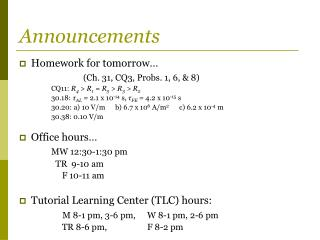DownloadDownload PresentationAnnouncements

Announcements

Télécharger la présentationAnnouncements

- - - - - - - - - - - - - - - - - - - - - - - - - - - E N D - - - - - - - - - - - - - - - - - - - - - - - - - - -
Presentation Transcript

1. Announcements • Homework for tomorrow… (Ch. 31, CQ3, Probs. 1, 6, & 8) CQ11: R4 > R1 = R5 > R3 > R2 30.18: τAL= 2.1 x 10-14 s, τFE= 4.2 x 10-15 s 30.20: a) 10 V/m b) 6.7 x 106 A/m2 c) 6.2 x 10-4 m 30.38: 0.10 V/m • Office hours… MW 12:30-1:30 pm TR 9-10 am F 10-11 am • Tutorial Learning Center (TLC) hours: M 8-1 pm, 3-6 pm, W 8-1 pm, 2-6 pm TR 8-6 pm, F 8-2 pm

2. Chapter 31 Fundamentals of Circuits (Kirchoff’s Laws and the Basic Circuit & Energy and Power)

3. Review… • Kirchoff’sjunction rule.. • Kirchoff’sloop rule…

4. The Basic Circuit Consider the basic circuit shown...

5. The Basic Circuit Consider the basic circuit shown... Notice: • NO junctions, SAME I everywhere. • Assuming ideal wires (R wire~0).

6. i.e. 31.1:Two resistors and two batteries Analyze the circuit shown in the figure. • Find the current in and the potential difference across each resistor. • Draw a graph showing how the potential changes around the circuit, starting from V = 0V at the negative terminal of the 6 V battery. 4 Ω 6 V 9 V 2Ω

7. Quiz Question 1 The potential difference across the 10 Ωresistor is… • 30 V. • 20 V. • 15 V. • 10 V. • 5 V.

8. Quiz Question 2 What things about the resistors in this circuit are the same for all three? • Current I. • Potential difference V. • Resistance R. • 1. and 2. • 2. and 3.

9. Quiz Question 3 Which light bulb is brighter? • Light bulb A. • Light bulb B. • Both are the same brightness.

10. 31.3:Energy and Power Current is NOT used up by the light bulb, ENERGY is!

11. 31.3:Energy and Power Current is NOT used up by the light bulb, ENERGY is! Q: What is the rate at which the battery supplies energy to the charges?

12. 31.3:Energy and Power Current is NOT used up by the light bulb, ENERGY is! Q: What is the rate at which the battery supplies energy to the charges? A: SI Units?

13. 31.3:Energy and Power Current is NOT used up by the light bulb, ENERGY is! Q: What is the rate at which the battery supplies energy to the charges? A: SI Units? [P] = J/s or W

14. i.e. 31.2:Delivering Power A 90 Ω load is connected to a 120V battery. How much power is delivered by the battery?

15. 31.3:Energy and Power Pbat is the energy transferred per second from the battery’s store of chemicals to the moving charges that make up the current.

16. 31.3:Energy and Power Pbat is the energy transferred per second from the battery’s store of chemicals to the moving charges that make up the current. Q: Where does that energy go?

17. 31.3:Energy and Power Pbat is the energy transferred per second from the battery’s store of chemicals to the moving charges that make up the current. Q: Where does that energy go? A: • The battery’s chemical energy is transferred to the thermal energy of the resistors. • The rate at which the battery supplies energy is exactly equal to the rate at which the resistor dissipates energy!

18. i.e. 31.3:The power of light How much current is “drawn” by a 100 W light bulb connected to a 120 V outlet?